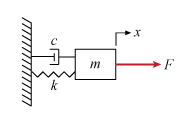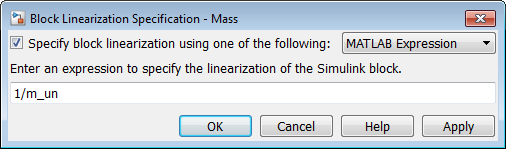## Model Uncertainty in Simulink for Robust Tuning

This example shows how to set up a Simulink® model for robust tuning against parameter uncertainty. Robust controller tuning or robust controller synthesis for a system modeled in Simulink requires linearizing the model such that the software takes parameter uncertainty into account. Doing so requires block substitution (Simulink Control Design) for linearization, to replace the value of blocks that have parameter uncertainty with uncertain parameters or systems.

In this example, you set up a model of a mass-spring-damper system for robust tuning, where the physical parameters of the system are uncertain. The example shows how to set up the model for robust tuning using software such as Control System Tuner or `systune` for `slTuner`. It also shows how to extract an uncertain system to use for robust controller design with `musyn`.

### Mass-Spring-Damper System

Open the Simulink model `rct_mass_spring_damper`.

`openExample('rct_mass_spring_damper')`This model represents a system for controlling the mass-spring damper system of the following illustration.In this system, the applied force F is the plant input. The PID controller generates the force necessary to control the mass position x. When the mass m, the damping constant c, and the spring constant k are fixed and known, tuning the PID coefficients for desired performance is straightforward. In practice, however, physical system parameters can be uncertain. You can use Control System Tuner or `systune` to tune the system robustly against the uncertainty, and achieve satisfactory performance within the range of expected values for these parameters.

### Specify Parameter Uncertainty

The model is configured to use the nominal or most probable values of the physical parameters, m = 3, c = 1, and k = 2. To tune the system against uncertainty in these parameters, specify the parameter uncertainty in the model.

First, create uncertain real (`ureal`) parameters for each of the three uncertainties. For this example, specify the uncertainty as a percentage variation from the nominal value.

```m_un = ureal('m',3,'Percentage',40); c_un = ureal('c',1,'Percentage',20); k_un = ureal('k',2,'Percentage',30);```

To specify these uncertainties in the model, use block substitution. Block substitution lets you specify the linearization of a particular block in a Simulink model. In the model, right-click the ```Spring Stiffness``` block in the model and select Linear Analysis > Specify Selected Block Linearization.In the Block Linearization Specification dialog box, check Specify block linearization using one of the following and enter `k_un` in the text field. Click OK.When you use Control System Tuner for this model, the software linearizes the model and tunes the tunable parameters using that linearization to compute system responses. Specifying `k_un` as the linearization of the `Spring Stiffness` block causes the software to use the uncertain parameter as the linearized value of the block instead of its nominal value, which is a constant, fixed gain of 2.

Because the uncertain parameters in this model, such as the spring stiffness, are implemented as scalar gain blocks, use a simple `ureal` parameter as the block substitution. For more complex blocks, construct a `uss` model that represents the uncertain value of the entire block.

Note

Use block substitution to specify the uncertainty of the block even if the block is an `Uncertain LTI System` block. Unless you explicitly specify the uncertain value as the block substitution, Control System Tuner and `slTuner` use the nominal value when linearizing `Uncertain LTI System` blocks.

In the same way, specify `c_un` as the block linearization for the `Damping` block. For the `Mass` block, in the Block Linearization Specification dialog box, enter `1/m_un` as the uncertain value, because the gain of this block is the inverse of the mass.### Tune With Control System Tuner

You can now open Control System Tuner for the model, create tuning goals, and tune the model. When you do so, Control System Tuner tunes the controller parameters to optimize performance over the entire range of uncertainty. Tuning-goal plots and response plots in Control System Tuner display multiple responses computed at random values of the uncertain parameters, as shown.This sampling provides a general sense of the range of possible responses, but does not necessarily reflect the true worst-case response.

### Configuration for `slTuner`

When you use `slTuner` for command-line tuning, you can specify uncertainties in the model using the Block Linearization Specification dialog box. Alternatively, you can specify the uncertain block substitutions without altering the model. To do so, use a block-substitution structure when you create the `slTuner` interface. For example, create a block-substitution structure for the `rct_mass_spring_damper` model.

```blocksubs(1).Name = 'rct_mass_spring_damper/Mass'; blocksubs(1).Value = 1/m_un; blocksubs(2).Name = 'rct_mass_spring_damper/Damping'; blocksubs(2).Value = c_un; blocksubs(3).Name = 'rct_mass_spring_damper/Spring Stiffness'; blocksubs(3).Value = k_un; ```

Use this structure to obtain an `slTuner` interface to the model with the uncertain values.

`UST0 = slTuner('rct_mass_spring_damper','Controller',blocksubs);`

You can now create tuning goals and tune the model. `systune` tunes the system to optimize performance over the entire range of uncertainty. For an example illustrating this robust-tuning workflow with `slTuner`, see Robust Tuning of Mass-Spring-Damper System.

### Extract `uss` Plant Model for Robust Controller Design with `musyn`

The `musyn` command synthesizes a robust controller for a plant assuming an LFT control configuration.Mapping this structure to the Simulink model,

• w is the reference input `r`, the output of the Step block.

• u is the control signal `F`, the output of the PID Controller block.

• z is the plant output `x`, the output of the Integrator block.

• y is the measurement signal, which is the controller input, or the output of the Sum block.

Use these signals with the `getIOTransfer` (Simulink Control Design) command to extract the plant P from the `slTuner` interface `UST0`. To do so, `UST0` must have analysis points defined at each of these locations. Examine the analysis points of `UST0`.

```getPoints(UST0) ```
```ans = 2×1 cell array {'rct_mass_spring_damper/Step/1[r]' } {'rct_mass_spring_damper/Integrator/1[x]'}```

There are already analysis points for w and z. Add the analysis points for u and y.

```addPoint(UST0,{'Sum1','Controller'}); getPoints(UST0)```
```ans = 4×1 cell array {'rct_mass_spring_damper/Step/1[r]' } {'rct_mass_spring_damper/Integrator/1[x]'} {'rct_mass_spring_damper/Sum1/1' } {'rct_mass_spring_damper/Controller/1[F]'} ```

You can now extract the plant model P for tuning with `musyn`. Use the analysis-point signal names, shown in brackets in the output of `getPoints`, to specify the inputs and outputs of P. For analysis points that do not have signal names, use the block name.

`Pg = getIOTransfer(UST0,{'r','F'},{'x','Sum'});`

`getIOTransfer` returns a `genss` model. In this case, because `Pg` excludes the controller block, `Pg` is a `genss` model with uncertain blocks only. Convert `Pg` to `uss` for controller design with `musyn`.

`P = uss(P)`
```P = Uncertain continuous-time state-space model with 2 outputs, 2 inputs, 3 states. The model uncertainty consists of the following blocks: c: Uncertain real, nominal = 1, variability = [-20,20]%, 1 occurrences k: Uncertain real, nominal = 2, variability = [-30,30]%, 1 occurrences m: Uncertain real, nominal = 3, variability = [-40,40]%, 1 occurrences Type "P.NominalValue" to see the nominal value, "get(P)" to see all properties, and "P.Uncertainty" to interact with the uncertain elements. ```

You can now use `musyn` to design a robust controller for P. For instance, to design an unstructured robust controller, note that P has one measurement signal and one control signal, and use the following command.

`[K,CLperf,info] = musyn(P,1,1);`

Alternatively, design a fixed-structure PID controller, as in the original Simulink model.

```C0 = tunablePID('K','PID'); CL0 = lft(P,C0); [CL,CLperf,info] = musyn(CL0);```

For more information about robust controller design, see `musyn`.